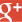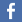﻿ Encrypt and Decrypt Vigenere cipher online ﻿

# Vigenere cipher Encrypt & Decrypt## Vigenere Cipher :

Vigenere cipher is a polyalphabetical cipher. In this kind of encryption, and unlike monoalphabetical ciphers (which are used in polyalphabetical ciphers though), one letter can be ciphered in different ways depending on its position in the text. Indeed, Vigenere cipher introduced the concept of key to encrypt the data. This key brings a huge evolution into ciphers, because it allows the one that uses it to resist (in a certain way) to letters frequency analysis.

The name Vigenere cipher comes from the diplomat Blaise de Vigenere who described this encryption (along with others) in 1586, in its book "Traité des Chiffres". However, similar encryption had already been described by Giovan Battista Bellaso. This cipher isn't exactly the same, as it first use a keyword to generate 5 alphabets, which will be used with a second key to encrypt the text.

The Vigenere encryption is very simple. We use a table, which is easy to remember in its original form. This table is bi-dimensionnal and have alphabet letters in X and Y axis (from A to Z). On X axis, the letters are the one from the plaintext that you want to encrypt. On the Y axis, the letters are the one from the key you want to use to encrypt the data. To encrypt, you start by repeating the key so that every letter from the plaintext has a corresponding key letter. That means that for a plaintext which has 7 characters, and a key that only have 3, you'll have to repeat 2 times the key, then just add th the first letter of the key. For instance, if we take the plaintext "Vigenere Cipher", with the key "key" :

Plaintext : Vigenere Cipher
Key : keykeyke ykeyke

You can see that the key has been repeated as much as necessary to fill all the plaintext length. One should notice how important it is to choose a long enough length for the key. It will improve the security of this cipher, as you see, if you choose a very short key, you'll have to repeat it more, and it will be easier for an attacker to find repetitions in your text (as we'll see later).

Once every letter from your plaintext have a corresponding key letter, you can encrypt the text using the table.

 Plaintext A B C D E F G H I J K L M N O P Q R S T U V W X Y Z K E Y A A B C D E F G H I J K L M N O P Q R S T U V W X Y Z B B C D E F G H I J K L M N O P Q R S T U V W X Y Z A C C D E F G H I J K L M N O P Q R S T U V W X Y Z A B D D E F G H I J K L M N O P Q R S T U V W X Y Z A B C E E F G H I J K L M N O P Q R S T U V W X Y Z A B C D F F G H I J K L M N O P Q R S T U V W X Y Z A B C D E G G H I J K L M N O P Q R S T U V W X Y Z A B C D E F H H I J K L M N O P Q R S T U V W X Y Z A B C D E F G I I J K L M N O P Q R S T U V W X Y Z A B C D E F G H J J K L M N O P Q R S T U V W X Y Z A B C D E F G H I K K L M N O P Q R S T U V W X Y Z A B C D E F G H I J L L M N O P Q R S T U V W X Y Z A B C D E F G H I J K M M N O P Q R S T U V W X Y Z A B C D E F G H I J K L N N O P Q R S T U V W X Y Z A B C D E F G H I J K L M O O P Q R S T U V W X Y Z A B C D E F G H I J K L M N P P Q R S T U V W X Y Z A B C D E F G H I J K L M N O Q Q R S T U V W X Y Z A B C D E F G H I J K L M N O P R R S T U V W X Y Z A B C D E F G H I J K L M N O P Q S S T U V W X Y Z A B C D E F G H I J K L M N O P Q R T T U V W X Y Z A B C D E F G H I J K L M N O P Q R S U U V W X Y Z A B C D E F G H I J K L M N O P Q R S T V V W X Y Z A B C D E F G H I J K L M N O P Q R S T U W W X Y Z A B C D E F G H I J K L M N O P Q R S T U V X X Y Z A B C D E F G H I J K L M N O P Q R S T U V W Y Y Z A B C D E F G H I J K L M N O P Q R S T U V W X Z Z A B C D E F G H I J K L M N O P Q R S T U V W X Y

We take the first letter from the plaintext, "V", the first letter from the key, "K", and we look in the table at which letter "V" and "K" cross. We can see that the first letter of encrypted text will be a "F". Then you just continue with each letter of the plaintext.
This will give us the encrypted text "fmeorcbi mmnripke" if we keep all the whitespaces (you can choose that on the options).

The "Table look" technique is easy for a human - slow but easy -, the best way to encrypt the text though will be to proceed by additions. If you look at the table you can notice that the encrypted letter's position in the alphabet is always equal to the key letter's position minus 1. For instance we had a "V" encrypted using a "K". K position is 11, if you add this minus 1 to the position of V in the alphabet (22), you will find F. When the addition is above 26, you start again at 1, that's a modulo 26 addition. For instance with our example, it would be (11 - 1) + 22 = 32, then modulo 26 addition : 32 % 26 = 6, and 6 is the position of "F" in the alphabet.

The encryption process follow exaclty the opposite way, in th table technique you just have to look for the key letter, "K", follow the line until you find the encrypted letter "F", then go up to the plaintext alphabet, to find the "V" letter. You could alos proceed by subtraction, instead of addition for encryption. For instance with our example, we take the encrypted letter "F", which position in the alphabet is 6, and the key-letter "K", which position is 11, and you substract : 6 - (11 - 1) = -4. If like here you obtain a negative result, just substract the absolute value to 26. for instance here to obtain the plaintext letter you just have to do 26 - 4 = 22, which is the position of "V".

### Cryptanalyse

Vigenere cipher is obviously not considered as secure since the Prussian Major Friedrich Kasiski broke it in 1863. Still it is interesting to analyze different ways to decrypt Vigenere cipher. First, it exists a case in which the security offered by Vigenere cipher is maximal, it is when the key length is exactly the same (or longer) than plaintext length. When the key is also randomly defined and is used only once, we use the name of one-time pad.

In most of the cases though, the key has a smaller length than the plaintext. In those cases, we use the Kasiski method (although Charles Babbage also successfully broke some Vigenere ciphers, we do not have any note from him so we can't know how he did). This method consists in finding repeated sub-strings into the encrypted text, that will allow the attacker to guess the key length. Once you find the key length, you can concatenate all the substrings to make a frequency analysis on it. If the text is long enough, it becomes possible to decrypt the cipher text. During the XXth, Friedman created a decryption method for polyalphabetical ciphers, including Vigenere cipher, this method is known as Index of Coincidence and allow to define if an encrypted text had been encrypted using monoalphabetical or polyalphabetical cipher, along to guess the key length.

The other decryption method, slower of course, is the bruteforce. To make this faster, you could observe the encrypted text to guess the key length. This will allow you to limit the possibilities for bruteforce (which consists in trying all different possibilities for a given length and a given alphabet). The key generally only consists on lower letters, which ease the task again.

To make the decryption more difficult, you also can create your own alphabet, or even add characters (numbers, punctuation, etc). The best way to secure Vigenere cipher is to choose a very long key. It was frequent when this cipher was still in use to choose entire chunks of a book to encrypt very important messages. The vigenere cipher shouldn't be used though since it isn't secure anymore. However it was used during three centuries without being broke.

﻿Mentions légales | 24,481,148 cracked hashes since 11/2015 | There are words in the database |